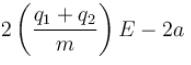Courses

# Electrostatics MCQ Level - 2

## 10 Questions MCQ Test Topic wise Tests for IIT JAM Physics | Electrostatics MCQ Level - 2

Description
This mock test of Electrostatics MCQ Level - 2 for Physics helps you for every Physics entrance exam. This contains 10 Multiple Choice Questions for Physics Electrostatics MCQ Level - 2 (mcq) to study with solutions a complete question bank. The solved questions answers in this Electrostatics MCQ Level - 2 quiz give you a good mix of easy questions and tough questions. Physics students definitely take this Electrostatics MCQ Level - 2 exercise for a better result in the exam. You can find other Electrostatics MCQ Level - 2 extra questions, long questions & short questions for Physics on EduRev as well by searching above.
QUESTION: 1

### The electric field inside a sphere which carries a volume charge density proportional to the distance from the origin ρ = α r, (α is a constant) is:

Solution:

We can consider all the charge inside the sphere to be concentrated on the center of sphere
Consider an elementary shell of radius x and thickness dx.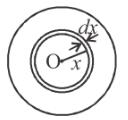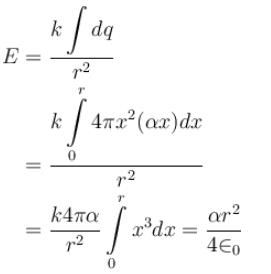The correct answer is: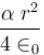QUESTION: 2

### Two concentric uniformly charged spheres of radius 10 cm and 20 cm are arranged as shown in the figure. Potential difference between the spheres is :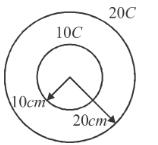Solution: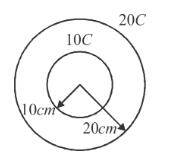Potential difference due to inner 10C charge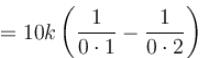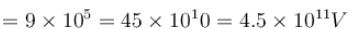Potential difference due to outer charge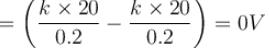∴ Potential difference =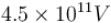The correct answer is: 4.5 × 1011 V

QUESTION: 3

### A neutral conducting spherical shell is kept near a charge q as shown. The potential at point P due to the induced charges is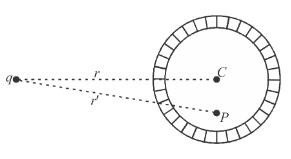Solution: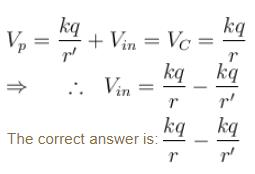QUESTION: 4

An infinitely long plate has surface charge density ρ. As shown in the figure, a point charge q is moved from A to B. Net work done by electric field is: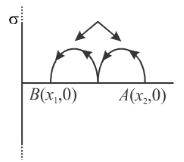Solution: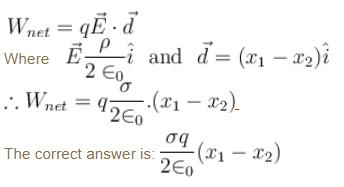QUESTION: 5

A dipole of dipole moment p is kept at the centre of a ring of radius R and charge Q. The dipole moment has direction along the axis of the ring. The resultant force on the ring due to the dipole is:

Solution:

Electric field due to ring at point P on its axis at a distance x from centre O of ring is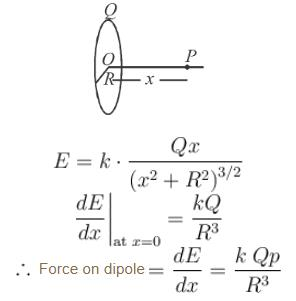The correct answer is: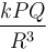QUESTION: 6

In the figure shown. A is a fixed charge. B (of mass m) is given a velocity v perpendicular to line AB. At this moment the radius of curvature of the resultant path of B is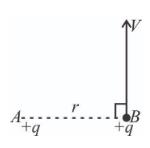Solution: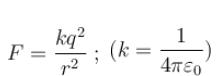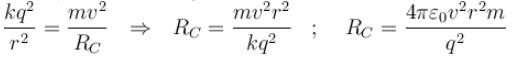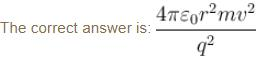QUESTION: 7

Figure shows three circular arcs, each of radius R and total charge as indicated. The net electric potential at the centre of curvature is :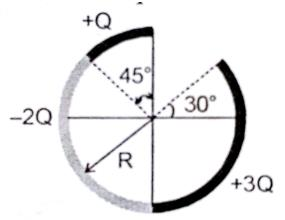Solution:

V = V1 + V2 + V3
(where  V1, V2  and  V3  are potentials due to the three parts of ring)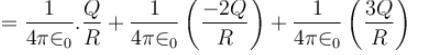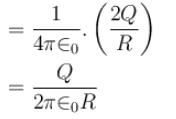The correct answer is: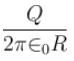QUESTION: 8

A conducting disc of radius R rotates about its axis with an angular velocity ω. Then the potential difference between the centre of the disc and its edge is (no magnetic field is present):

Solution: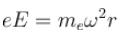(Balancing of forces of e–)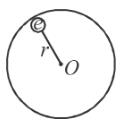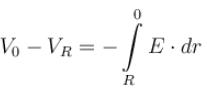Also,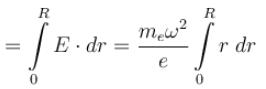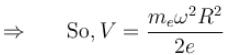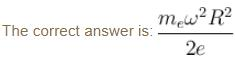QUESTION: 9

Two identical small balls each have a mass m and charge q. When placed in a hemispherical bowl of radius R with friction less, non-conducting walls, the beads move and at equilibrium, the line joining the balls is horizontal and the distance between them is R (figure). Neglect any induced charge on the hemispherical bowl. Then the charge on each bead is: (here,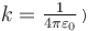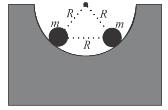Solution:

The bowl exerts a normal force N on each bead, directed along the radial line or at 60° above the horizontal. Consider the free-body diagram of the bead on the left with the electric force Fe  applied.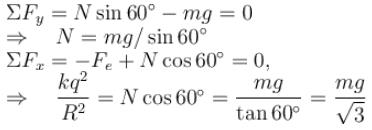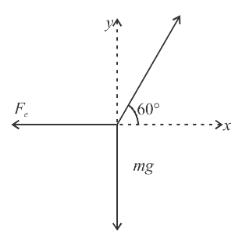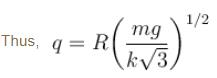The correct answer is: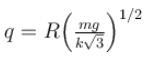QUESTION: 10

Two positively charged particles of charges q1 and q2 have mass m each. A uniform electric field having magnitude E exists in positive x direction as shown in the figure. The given two charged particles are released from rest at t = 0 as shown in the figure. If position of q1 at t = 2sec is given by coordinate (+2a, 0) then the x-coordinate of q2 at t = 2sec is (neglect gravitational interaction between the particles)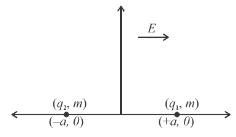Solution:

The acceleration of centre of mass of system of particles is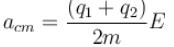∴   x-coordinate of centre of mass at t = 2 second is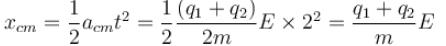Let the x-coordinates of q1 and q2 at t = 2 sec be x1 and x2 ; [x1 = 2a at t = 2 sec.]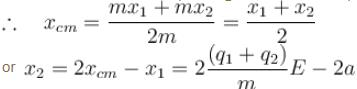The correct answer is: